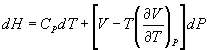5HC - October 1997

Part A (20 x 2 = 40 Marks)

1. A centrifugal fan forms _______ system
2. Zeroth law of thermodynamics defines _______
3. The ratio of specific heat of a gas at constant pressure and at constant volume always varies with _______
4. Internal energy of ideal gas is a function of _______ alone
5. Carnot cycle comprises _______ isothermal process and two _______ process
6. A heat engine is supplied with 300 kJ/sec of heat at 600 K and heat rejection takes place at 300 K. Heat rejected is 100 kJ/sec. The data refers to _______ cycle
7. Value of extensive properties of a system depends on _______ of the system
8. Heat and work are _______ functions
9. For a higher value of polytropic index the compressor work for a given pressure ratio is _______
10. Enthalpy of a gas depends on _______
11. Inter-cooling in an air compressor helps in _______
12. The function of providing clearance volume in a reciprocating air compressor is _______
13. One ton of refrigeration is _______
14. The refrigerant used in household refrigerator is _______
15. The entropy of the universe _______
16. The application of Gibbs Duhem equation is _______
17. The compressibility factor of an ideal gas is _______
18. What is fugacity coefficient?
19. Define Activity
20. Define equilibrium constant
21.

22. (a) What is the scope and limitations of thermodynamics? (6)
23. (b) Explain

(i) System and Surroundings (3)

(ii) Macroscopic and Microscopic aspects (3)

Or

(c) Explain the work required in the case of Isothermal and Adiabatic process (8)

(d) Calculate the work done when 65.38 gram of zinc dissolves in HCl (i) in a open beaker (ii) a closed beaker at 300 K (4)

24. (a) Explain compressibility factor (5)
25. (b) Derive van der Waal's equation of stae (7)

Or

(c) Show that in multistage compression for minimum work, the inter-stage pressure is the geometric mean of the initial and final pressures (8)

(d) Methane is to be compressed in an adiabatic compressor with inter-stage cooling from an initial pressure of 1.03 x 105 N/m2 to 201.06 x 105 N/m2. If the number of stages in the compressor is 4, what would be the compression ratio for optimal operation? (4)

26. (a) Show that CP - CV = R for an ideal gas
27. (b) Show thatOr

(c) What is chemical potential? (3)

(d) Explain (i) Helmholtz free energy (ii) Gibbs free energy (iii) Joule Thomson Coefficient (3 x 3 = 9)

28. (a) Derive the coexistence equation (8)
29. (b) For a binary mixture of 'A' and 'B' activity coefficient data for 'A' are available over the entire composition range, while one data is available for 'B'. Show how you would determine activity coefficient for 'B' over the entire range of composition (4)

Or

(c) Explain equilibrium curves and B.P. diagrams (6)

(d) An experimental determination of a VLE state for the ethanol, toluene system gave the following results:

Standard V.P. at 45oC, Ethanol = 173 mm Hg, Toluene = 75.4 mm Hg, x1 = 0.3; y1 = 0.634; PT = 183 mm Hg. Calculate (i) the liquid phase activity coefficient (ii) does the liquid phase exhibit positive or negative deviation from the ideal solution behavior? (6)

30. (a) What is the effect of pressure on equilibrium constant? (6)
31. (b) Compute the equilibrium constant for the water gas reaction at 540oC (6)

CO + H2O à CO2 + H2

Data:

 DHf cal/gmol CO -26,420 H2O -57,800 CO2 -94,050 H2 0

Or

(c) For the reaction SO2 + 1/2 O2 à SO3 in equilibrium at 900 K, what pressure is required for a 90% conversion of SO2 if the initial mixture is equimolar in the reactants. Take Ka = 1.3 at 900 K.